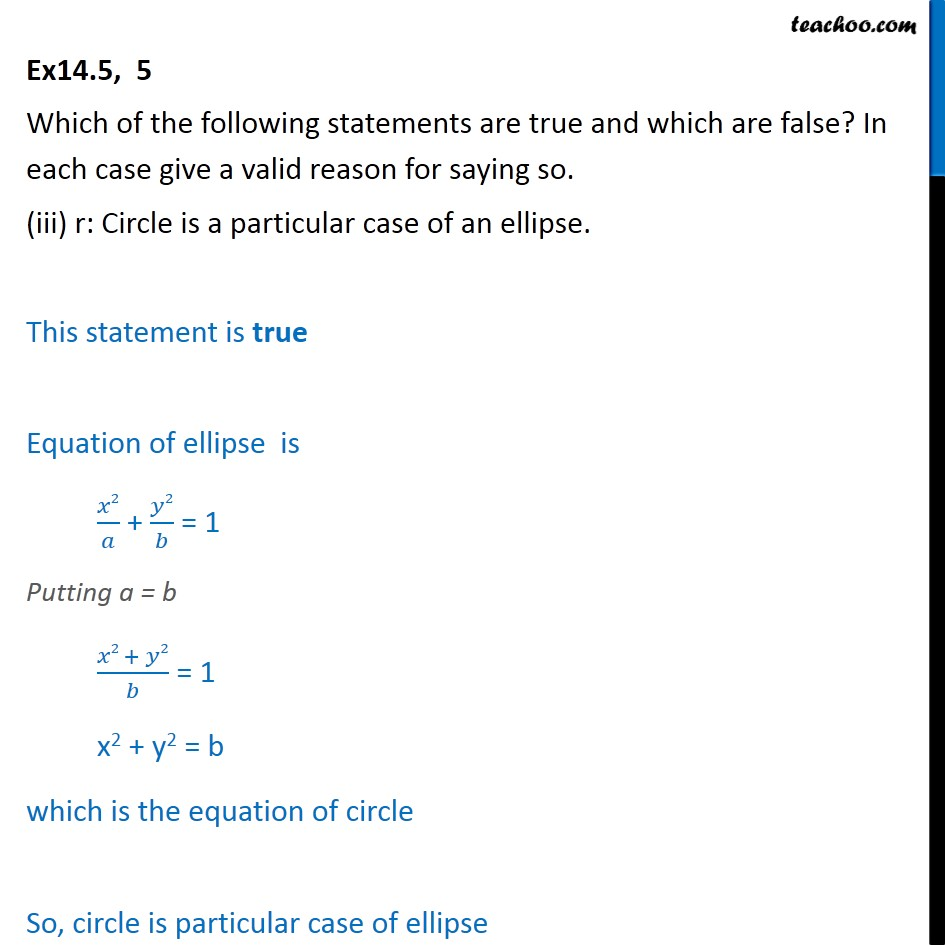Proving Statements true or false

Mathematical Reasoning
Serial order wiseLearn in your speed, with individual attention - Teachoo Maths 1-on-1 Class

### Transcript

Ex14.5, 5 Which of the following statements are true and which are false? In each case give a valid reason for saying so. (iii) r: Circle is a particular case of an ellipse. This statement is true Equation of ellipse is ﷐𝑥2﷮𝑎﷯ + ﷐𝑦2﷮𝑏﷯ = 1 Putting a = b ﷐𝑥2 + 𝑦2﷮𝑏﷯ = 1 x2 + y2 = b which is the equation of circle So, circle is particular case of ellipse